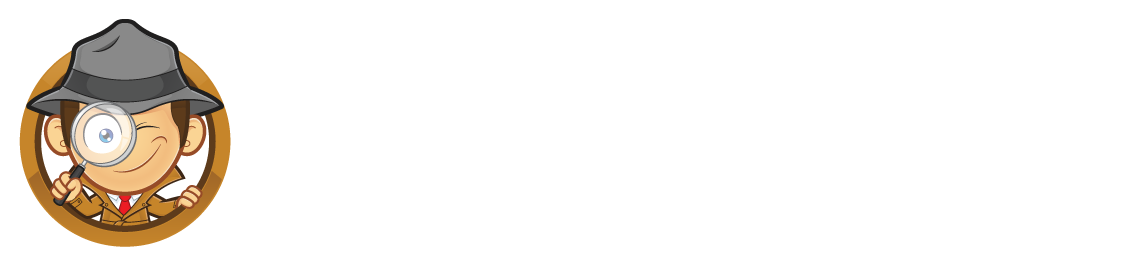Learning to spell numbers is a lot of fun. Sometimes it’s a bit tricky. We have to look really closely, just like a detective!Look at the spelling of these numbers and try to remember them.

• 1 = one
• 2 = two
• 3 = three
• 4 = four
• 5 = five
• 6 = six
• 7= seven
• 8 = eight
• 9 = nine
• 10 = ten
• 11 = eleven
• 12 = twelve
• 13 = thirteen
• 14 = fourteen
• 15 = fifteen
• 16 = sixteen
• 17 = seventeen
• 18 = eighteen
• 19 = nineteen
• 20 = twenty